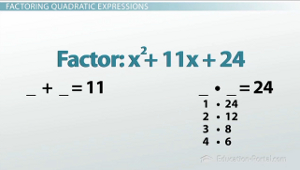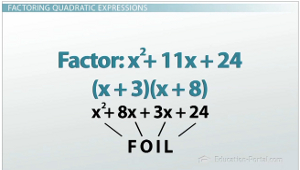# How to Factor Quadratic Equations: FOIL in Reverse

An error occurred trying to load this video.

Try refreshing the page, or contact customer support.

Coming up next: Factoring Quadratic Equations: Polynomial Problems with a Non-1 Leading Coefficient

### You're on a roll. Keep up the good work!

Replay
Your next lesson will play in 10 seconds
• 0:04 3rd Grade Review
• 1:25 Multiplying Binomials
• 2:48 Factoring Quadratic…
• 8:09 Lesson Summary

Want to watch this again later?

Timeline
Autoplay
Autoplay
Speed

#### Recommended Lessons and Courses for You

Lesson Transcript
Instructor: Luke Winspur

Luke has taught high school algebra and geometry, college calculus, and has a master's degree in education.

So, you know how to multiply binomials with the FOIL method, but can you do it backwards? That's exactly what factoring is, and it can be pretty tricky. Check out this lesson to learn a method that will allow you to factor quadratic trinomials with a leading coefficient of 1.

## Third Grade Review

Probably the most fundamental concept of algebra is the use of a variable (like x) to represent any number we want. That means that a lot of the stuff we do in an algebra class is actually stuff you learn in elementary school; we just use variables instead of specific numbers. I've found that one of the best ways to teach algebra is to first review the skill with specific numbers and then apply that same skill to our new algebraic problem.

What is 3 * 5? I think I need my calculator for this one. Ah! 15. Okay. Another third grade question, but this one might actually stump you because you'll need to remember some vocabulary: factor 15. So, the question really becomes, what does it mean to factor something? Turns out that it simply means to split that number up into what you can multiply to get it. So when I factor 15, I just turn it into 3 * 5. That means factoring is just doing multiplication in reverse. A little more vocab before we move on here. In the statement 3 * 5 = 15, 3 and 5 are called the factors of 15, while the answer to a multiplication problem is called the product.

## Multiplying BinomialsSo, back to algebra class. Let's do the same set of problems that we just did - but with variables this time around. So, instead of 3 * 5, how about (x + 3)(x + 5)?

There are a lot of different ways to multiply binomials just like this, but the most well-known way is the FOIL method. FOIL is an acronym that helps us remember to multiply the first terms, the outer terms, inner terms and finally the last terms. Once we've done that, we can combine our like terms to get our answer, x2 + 8x + 15.

Following the same order of questions from the third grade review, the next thing I'll ask is this: factor x2 + 8x + 15. We now know that this means to break up our number into the two things that I can multiply to get it (its two factors). Since we just did that, I know that the answer is going to be (x + 3)(x + 5), but what if we hadn't just done it? How would we know exactly how to break this trinomial up into its two separate binomials?

This question is what factoring quadratic expressions is all about, and it can be pretty tricky. But if we look closely at the example we just did, try to find some patterns and then generalize those patterns, we'll be able to come up with a method that will help you factor most quadratic trinomials you'll encounter.

## Factoring Quadratic Expressions

Let's look closely at that last problem we just did. We said we could factor x2 + 8x + 15 as (x + 3)(x + 5). What do the numbers from the trinomial (the product) have to do with the numbers from the two binomials (the factors)? Two things, actually!

3 + 5 gives us 8, which was the coefficient from the middle term from the trinomial. And 3 * 5 gives us 15, the constant on the end of the trinomial. This means that if you can find two numbers that add to the middle term of your trinomial and multiply to the constant on the end, those are going to be the two numbers in your factored expression.Let's see if we can apply this idea to a different problem; maybe factor x2 + 11x + 24. So, for our method to work, we need to find two numbers that add up to the middle coefficient (11) and that will also multiply to the constant on the end (24). Sometimes the answer is going to be obvious, but sometimes it will be hard to see. When it's hard to see right away, I like to write out all the different ways we can multiply to get the constant on the end and then see which one of those will add up to the number in the middle. Let's do that here to practice. We will always be able to do 1 times the number. In this case, (1 * 24). 24 is even, so 2 goes into it as well, so (2 x 12), I think 3, (3 x 8) and 4, (4 x 6), and that's it. I could do the other ones, (6 * 4), (0 * 3), but those are just the same, backwards, so those don't matter. So we have these four options - which ones add up to 11? Hey, 3 and 8!

That means that x2 + 11x + 24 factors into (x + 3)(x + 8). If you'd like to check your answer, you can quickly multiply it out and make sure it ends up back where we started! If we do that here, sure enough, we're all good!

To unlock this lesson you must be a Study.com Member.

### Register to view this lesson

Are you a student or a teacher?

### Unlock Your Education

#### See for yourself why 30 million people use Study.com

##### Become a Study.com member and start learning now.
Back
What teachers are saying about Study.com

### Earning College Credit

Did you know… We have over 160 college courses that prepare you to earn credit by exam that is accepted by over 1,500 colleges and universities. You can test out of the first two years of college and save thousands off your degree. Anyone can earn credit-by-exam regardless of age or education level.